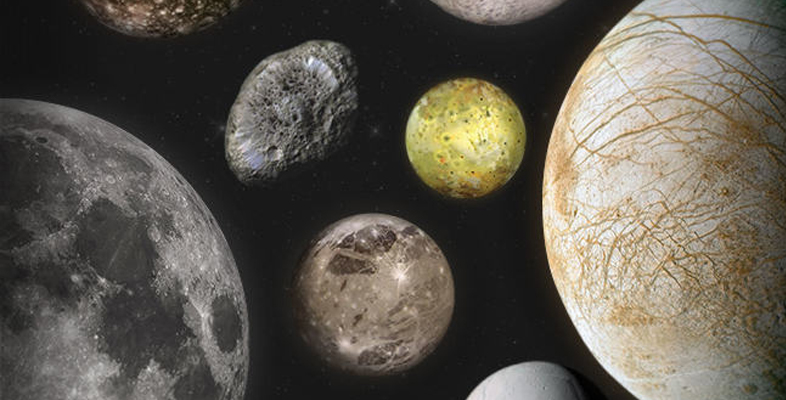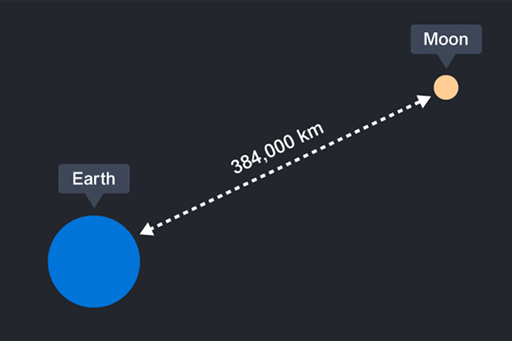Science, Maths & Technology

### Become an OU studentMoons of our Solar System

Start this free course now. Just create an account and sign in. Enrol and complete the course for a free statement of participation or digital badge if available.

# 1.3 The Moon – some facts

The Moon is about 4.5 billion years old. Current thought is that it was most likely formed by a giant impact, as you saw in Week 1.

The distance to the Moon is known accurately due to reflectors that were placed on it during the Apollo missions. The reflectors, part of the Lunar Laser Ranging Experiment, allow lasers to be shone onto the Moon and the time taken for the laser light to get there and back can be measured. It is then possible, using a simple equation, to calculate the distance to the Moon. You can watch a four minute video of Brian Cox explaining just this [Tip: hold Ctrl and click a link to open it in a new tab. (Hide tip)] .Figure 4 Distance between the Earth and the Moon.

Next, you have a chance to try calculating the distance to the Moon for yourself. It’s a very basic non-scored quiz. Don’t worry if you don’t know some of the answers.

## Activity 1 Calculating the distance to the Moon

Timing: Allow approximately 15 minutes.

Which is the correct equation for calculating the distance to the Moon? Note that ‘time’ refers to the time a laser pulse takes to get to the Moon and back to Earth.

a.

distance = (time/speed)/2

b.

distance = (speed × time)/2

c.

distance = (speed/time)/2

b.

This is correct because speed = distance/time. Multiplying both sides by time gives: distance = speed × time. Then speed × time is divided by 2 because the time obtained by shining the laser is the time for the light to go to the Moon and back to Earth, whereas all you want to know is the time for the light to reach the Moon.

What is the speed of the laser light?

a.

The speed of light

b.

The speed of sound

c.

It is different depending on the laser

a.

This is correct. Since a laser is simply a light source so laser light travels at the speed of light, independent of the frequency of the laser.

What is the distance to the Moon?

a.

~770 000 km

b.

~260 000 km

c.

~390 000 km